# Faster R-CNN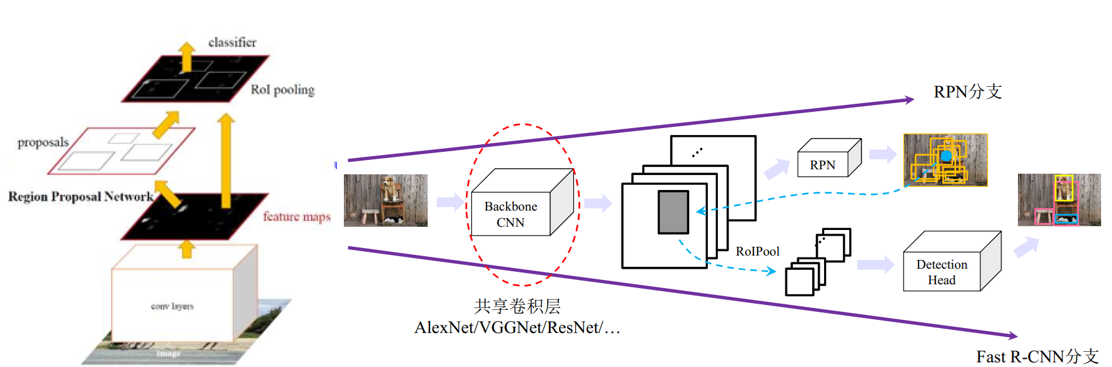# Region Proposal Layer

Region Proposal Layer包括RPN和3个layers – Proposal Layer, Anchor Target Layer and Proposal Target Layer.

## RPN（Region Proposal Network）

Anchor实际上为不同尺度的矩形框，用来框出原始图像中的目标，具有scale和ratio两个参数。作者使用了3个scale：{128,256,512}和3和ratio：{1:1，1:2，2:1}，这些框基本满足了原始图像的不同目标。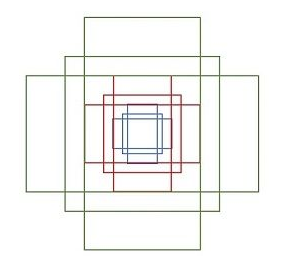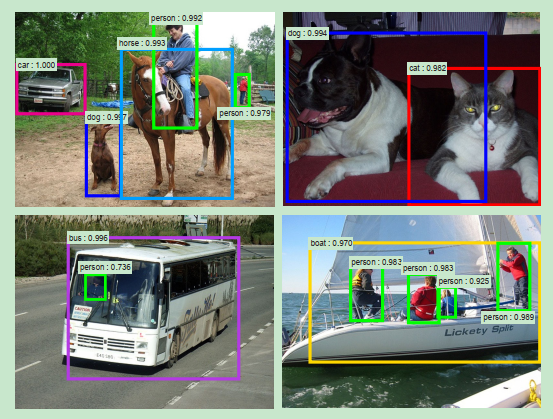9种anchors只是初始的检测框，后面的bounding box regression会会对检测框进行微调。下面展示了对于给定3D的feature map得到进行分类和回归检测框：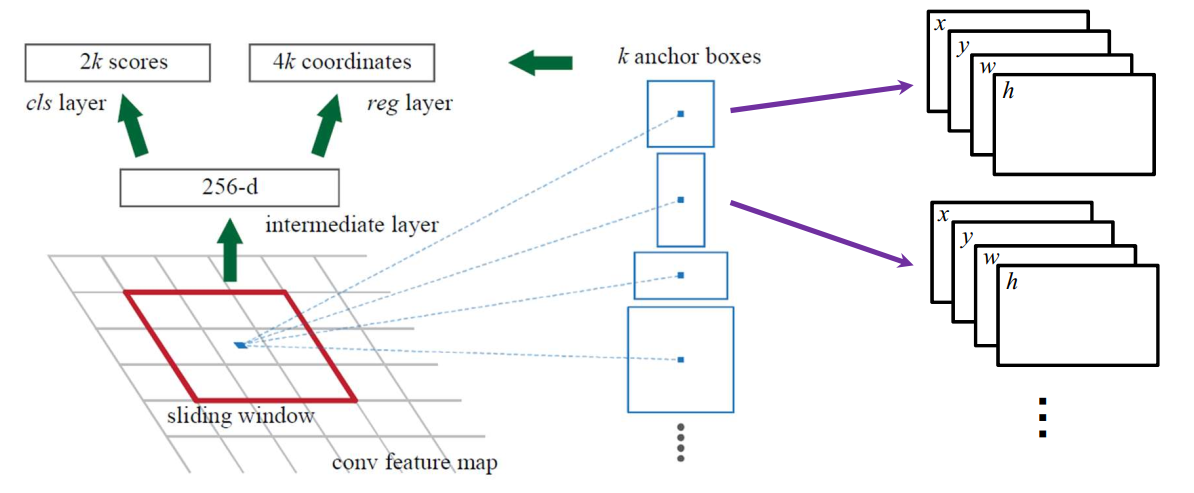## Proposal Layer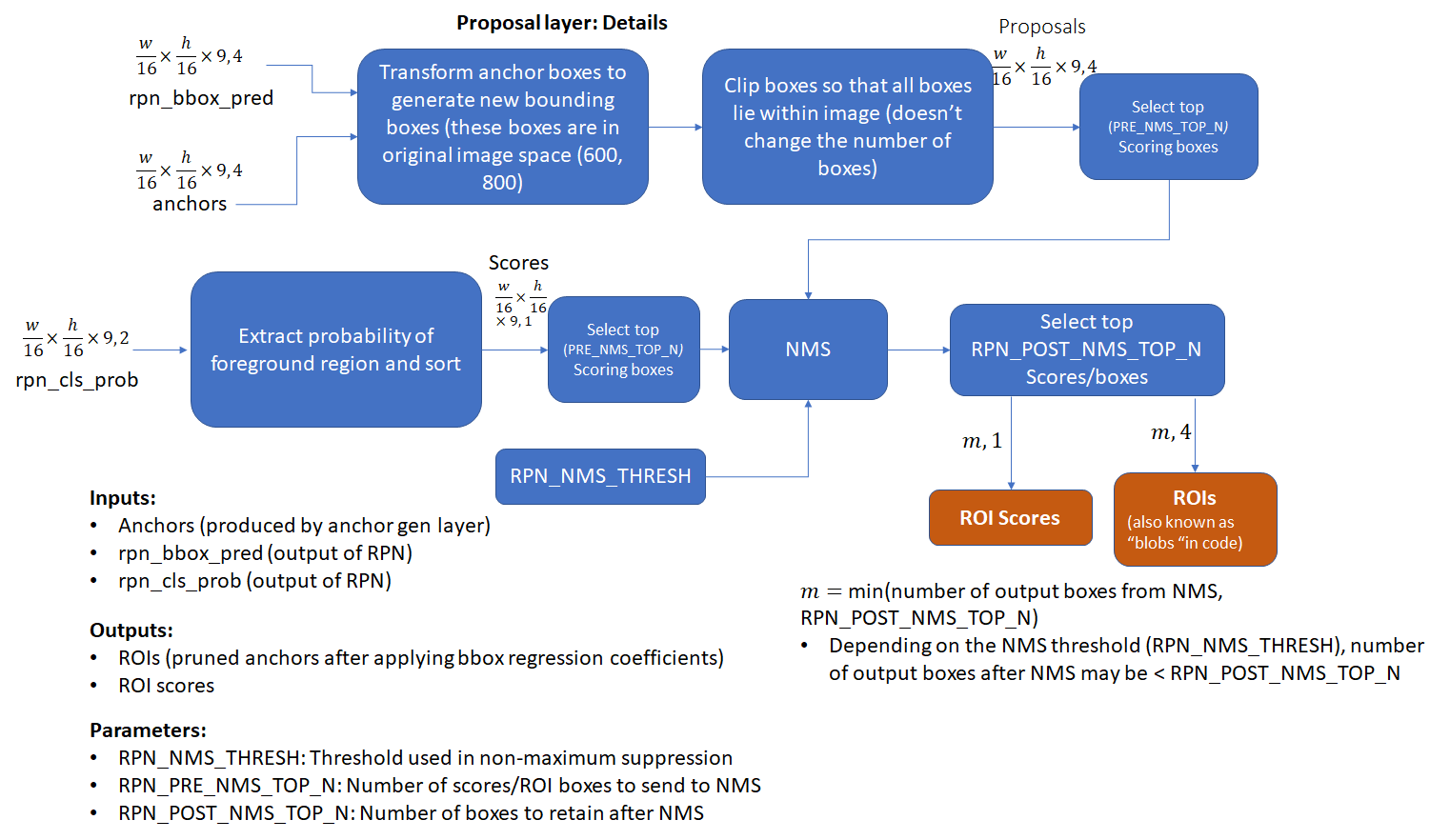## Anchor Target Layer

• anchor 与任一目标的 ground truth box 的IoU值最大
• anchor 与任一目标的 ground truth box 的IoU值 > 0.7

RPN 网络的 loss 函数同样是一个多任务的loss，包含两个部分，classification 的 loss 和 regression 的 loss：
L ( { p i , t t } ) i = 1 , 2 , . . . , N = 1 N c l s ∑ i L c l s ( p i , p i ′ ) + λ 1 N r e g ∑ i L r e g ( t i , t i ′ ) L(\{p_{i},t_{t}\})_{i=1,2,...,N}=\frac{1}{N_{cls}}\sum_{i}L_{cls}(p_{i},p_{i}')+\lambda\frac{1}{N_{reg}}\sum_{i}L_{reg}(t_{i},t_{i}')
i i 是一个 mini-batch 下 anchor 的索引。 p i p_{i} 是 anchor 为目标的概率。当anchor为目标时， p i ′ p_i' 为1，否则为0。 t i t_i 是预测框的位置坐标， t i ′ t_i' 是ground truth的坐标。 N c l s N_{cls} 为mini-batch的大小（如 N c l s N_{cls} = 256）， N r e g N_{reg} 为anchor的数量（如 N c l s N_{cls} ~ 2400),作者在实验中验证 λ \lambda 为10效果最好。
L c l s ( p i , p i ∗ ) = − log ⁡ [ p i ∗ p i ′ + ( 1 − p i ) ( 1 − p i ′ ) ] L r e g ( t i , t i ′ ) = s m o o t h _ l 1 _ l o s s ( t i − t i ′ ) L_{cls}(p_{i},p_{i}^{*})=-\log[p_{i}*p_{i}'+(1-p_{i})(1-p_{i}')]\\ L_{reg}(t_{i},t_{i}')=smooth\_l1\_loss(t_{i}-t_{i}')

# 采用交替训练(Alternating Train)

（1）使用预训练的Backbone训练RPN，并finetune backbone。
（2）用基于上一步得到的RPN生成的proposal来训练Faster R-CNN，并finetune backbone。
（3）固定共享的卷积层Backbone，训练PRN。
（4）固定共享的卷积层，基于上一步得到的RPN生成的proposal来训练训练Fast R-CNN

10-0405-139万+
05-132335
05-06940
02-211770
03-042万+
11-079708
04-18540
11-021412
10-247126
03-08984
10-30213
08-27
01-14201
07-132万+
09-291万+
12-19953
04-052229
©️2020 CSDN 皮肤主题: 编程工作室 设计师:CSDN官方博客